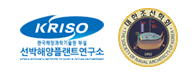Instructions to participants
Case 5.2: ONRT, trajectories

# Case 5.2: ONRT

## 1. Description of case 5.2

• Free running at model point in calm water (mandatory)
• With propellers and rudders
• With bilge keels
• LPP = 3.147 [m], (model scale 1:48.935)
• Radius of gyration for yaw and pitch 0.246LPP (in air)
• Radius of gyration for roll 0.344B (in air)
• GM=2.068 [m] in full-scale (0.0423 [m] in model)
• Approach speed U0 = 15.1 [kn] in full-scale (1.11 [m/s] in model)
• Re∗=3.39×106, Fr∗=0.20, g = 9.80665 [m/s2]
• Rudder rate 5.0 [deg/s] in full-scale (35.0 [deg/s] in model)
• Water depth: Infinity
Re∗: Nominal Reynolds number, the Reynolds number when ship runs in calm water with constant propeller rate of revolution.
Fr∗: Nominal Froude number, the Froude number when ship runs in calm water with constant propeller rate of revolution.
All quantities are non-dimensionalized by desired approach speed (U0), length between perpendiculars (LPP):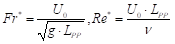(1)
where g is the gravitational acceleration and ν is the kinematic viscosity.

## 2. Experimental data

The submissions in case 5.2 will be compared to model tests carried out by IIHR. Roll decay result will be provided: time series of roll angle versus time.

## 3. Requested computations

The requested info are 3 submissions. You have to do 5.2.1 before to submit 5.2.2, you have to submit 5.2.2 before to submit 5.2.3.

##### Table 1: Requested computations
Manoeuvre Starting speed
5.2.1 Self propulsion information Fr = 0.20 (equivalent to 15.1 knots full scale)
5.2.2 20°/20° zigzag test, starting to starboard side Fr = 0.20 (equivalent to 15.1 knots full scale)
5.2.3 35° turning circle test, starting to portside Fr = 0.20 (equivalent to 15.1 knots full scale)

## 3.1 Submission 5.2.1: Self-propulsion

This submission is especially interesting for simulations based on URANS CFD. This case shows the “neutral rudder angle”, and the obtained longitudinal equilibrium (the propeller rpm and thrust). For simulations based on coefficient models, the neutral angle will often be zero.
1. All results are to be given for model scale conditions.
2. All simulations results should be provided in the format described in section 4.
3. The propeller rate of revolution should correspond to the self-propulsion point of the model. When the RPM is unknown (because for example a constant thrust or actuator disk model is used in CFD based simulations) please indicate this.
4. Similar to the experiment, the rudders should be controlled by following autopilot during course keeping: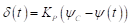(2)
where δ(t) is rudder angle, proportional gain KP is 1.0, ψC is the target yaw angle and ψ(t) is yaw angle. The maximum rudder rate should be assigned to 35.0 [deg/s]. Rudder angle should be controlled by P controller during approaching before starting rudder execution for manoeuvring (Target yaw angle: ψC=0°)
Please see section 6 for an example of the submission.

## 3.2 Submission 5.2.2: 20°/20° starboard side zigzag manoeuvre

1. All results are to be given for model scale conditions.
2. All simulations results should be provided in the format described in section 4.
3. The propeller rate of revolution should correspond to the self-propulsion point of the model. When the RPM is unknown (because for example a constant thrust or actuator disk model is used in CFD based simulations) please indicate this.
4. Similar to the experiment, the rudders should be controlled by Equation (2) during approaching before starting rudder execution for starboard side zigzag.
5. During the zigzag manoeuvre, the rudders should go to the real geometric 20 degrees (not to 20 degrees away from the neutral angle).
Please see section 5 for an example of the submission.

## 3.3 Submission 5.2.3: 35° portside turning circle manoeuvre

1. All calculations are to be conducted for model scale conditions.
2. All simulations results should be provided in the format described in section 4.
3. The propeller rate of revolution should correspond to the self-propulsion point of the model. When the RPM is unknown (because for example a constant thrust or actuator disk model is used in CFD based simulations) please indicate this.
4. Similar to the experiment, the rudders should be controlled by Equation (2) during approaching before starting rudder execution for starboard side zigzag.
5. During the turning circle manoeuvre, the rudders should go to the real geometric 35 degrees (not 35 degrees away from the neutral angle).
Please see section 7 for an example of the submission.

## 4. Format

1. The trajectory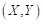and time histories of heave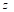¸ angular motions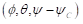, dimensional velocities for 6DOF motions (u,v,w,p,q,r), rudder angle δ, thrust coefficient T and torque coefficient Q for each propeller and propeller rate of revolution n [rps] after the ship was released (t = t0), should be submitted.
2. The ship position or trajectory should be given in an Earth-fixed coordinate system with X pointing North, Y pointing East, and Z pointing downward as shown in Figure 1. This XY axis system remains horizontal in the earth. The ship was released at (X0, Y0) when t = t0 . The roll angle (ϕ) is positive for pushing starboard into the water, pitch (θ) is positive for bow up position and yaw angle (ψ) ) is positive for bow turned to starboard. The reported trajectory is dimensional and angular motions should be reported in degrees. The angular velocities should be reported in degree second. The reported yaw angle should be the deviation of yaw angle respect to the target yaw i.e. ψ-ψC.
3. All velocities for 6DOF motions (u, v, w, p, q, r) should be reported in ship-fixed coordinate system with x axis positive toward bow, y axis positive toward starboard and z axis positive downward. This xyz axis system rotates with the ship in yaw but not pitch and roll, i.e., remains horizontal in the earth. It is a so-called “yawed-only reference system”. Given this convention, the reported velocities are: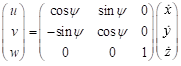(3)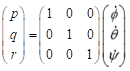(4)
In Equation (4), the unit of p, q, r,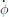,and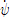is [deg/s].
4. Rudder angle (δ) is positive when trailing edge moves to portside.
5. The thrust T and torque Q for each propeller should be reported in shaft coordinate system with x axis positive toward the engine. Values are given dimensional
6. All motions should be reported at O. The origin is located on O which is located at midships, the intersection of ordinate 10 the centre line plane and the still water waterline.
Time series of the manoeuvres should be send to the organizers in an excel file. The data shall be give on model scale, and it will be compared to model scale data.
The submission consists of an excel file (example is given) with 19 columns and with rows for every time step. The time step is free to chose, but a small enough time step somewhere around 0.05 to 0.01 is recommended to get sufficient accuracy. The following quantities are asked:

##### Table 2: Submission variables
Symbol Item Unit
t time s
X X position in earth fixed system m
Y Y position in earth fixed system m
Z Z position in earth fixed system m
ϕ Roll angle degrees
θ Pitch angle degrees
ψ Yaw angle degree
u surge velocity m/s
v sway velocity m/s
w heave velocity m/s
p roll rate deg/s
q pitch rate deg/s
r yaw rate deg/s
δ Rudder angle deg
n Propeller revolutions of portside propeller rpm
TPS Thrust of portside propeller N
TSB Thrust of starboard side propeller N
QPS Torque of portside propeller Nm
QSB Torque of starboard side propeller Nm
These data are illustrated in Figure 1. The origin is located on O. The time t=0 is the time when the steering starts. The initial direction of the model is the direction of X-axis. Initial conditions at t = 0 are: X0=0, Y0=0, u=U0, δ=δ0 (which is the neutral rudder angle)
There is a right-handed earth-fixed coordinate system x0y0z0, and a horizontally moving right handed body-fixed coordinate system xyz. The body-fixed coordinate system has its origin in O (midship), and the xy plane moves with the ship. The heading angle is defined as the angle between X- and x0-axis. u and v are the velocity components in x and y direction. the hull drift angle will be defined as β=atan(-v/u), and the total velocity U=√(u2+v2).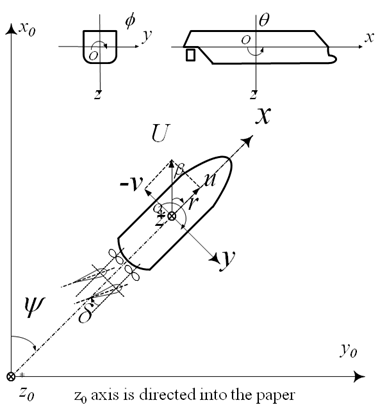Figure 1: Illustration of the sign convention of the earth-fixed and body-fixed coordinate system.

## 5. Elaborated example for test case 5.2.1

For ONRT, we elaborated an actual example of an actual submission compared to actual experimental data. For case 5.2.1, the comparison will look as displayed in the following figure. On top of the time traces as presented in below Figure 2, the average of the time traces will be determined. The averages are the comparison variables. For the time trace of Figure 3, these averages are given. In the case of unsteady , time domain RANS, these values will be asked. For the case of coefficient based simulations, or simulations based on empirical models, these average values can be given as 1 and 0.

##### Table 3: EFD values summary for Case 5.2.1
Variable Value
Z[m] 2.093E-03
ϕ[deg] 2.952E-02
θ[deg] -4.134E-02
ψ[deg] -1.724E-01
u[m/s] 1.128E+00
v[m/s] 4.277E-03
w[m/s] 2.500E-05
p[deg/s] 1.585E-03
q[deg/s] 1.714E-03
r[deg/s] 1.506E-02
δ[deg] 1.937E-01
n[rpm] 5.382E+02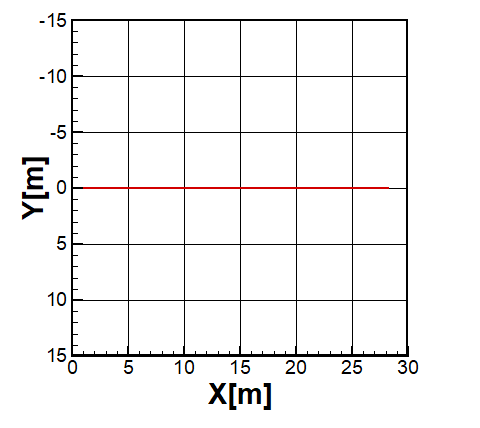Figure 2: Example of trajectory of Case 5.2.1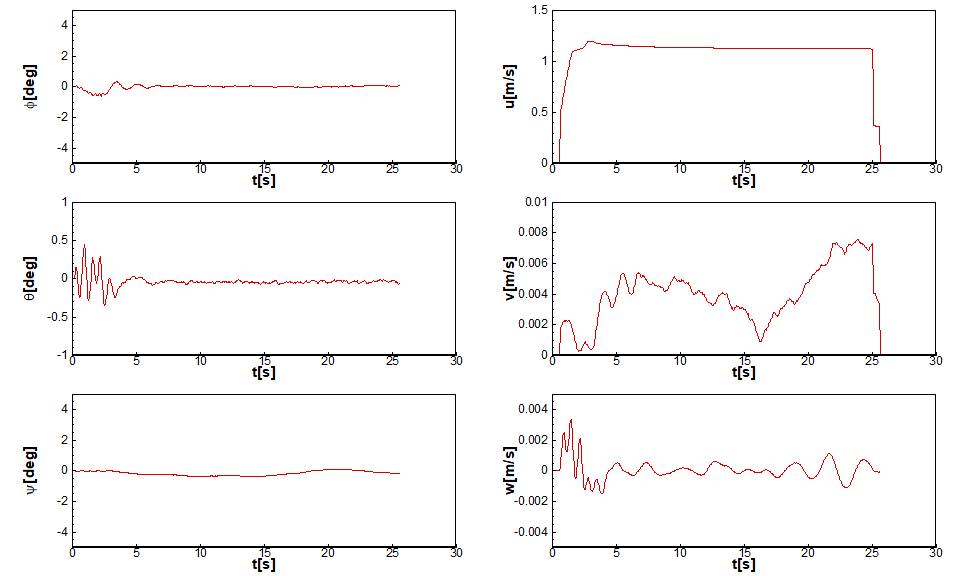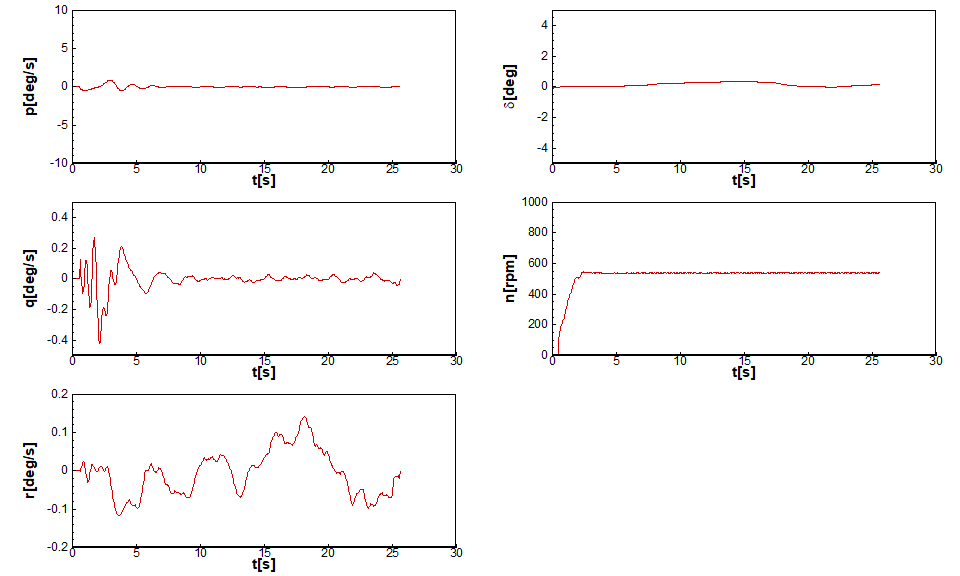Figure 3: Example of time traces of Case 5.2.1.

## 6. Elaborated example for case 5.2.2: 20°/20° starboard side zigzag

For case 5.2.2, the time traces will be plotted for comparison and this will look as displayed in Figure 5. From these time traces, the typical characteristics will be determined. These characteristics will be the comparison variables. Table 4 gives these characteristics. The bold variables are the primary validation variable, the non-bold are the secondary validation variables.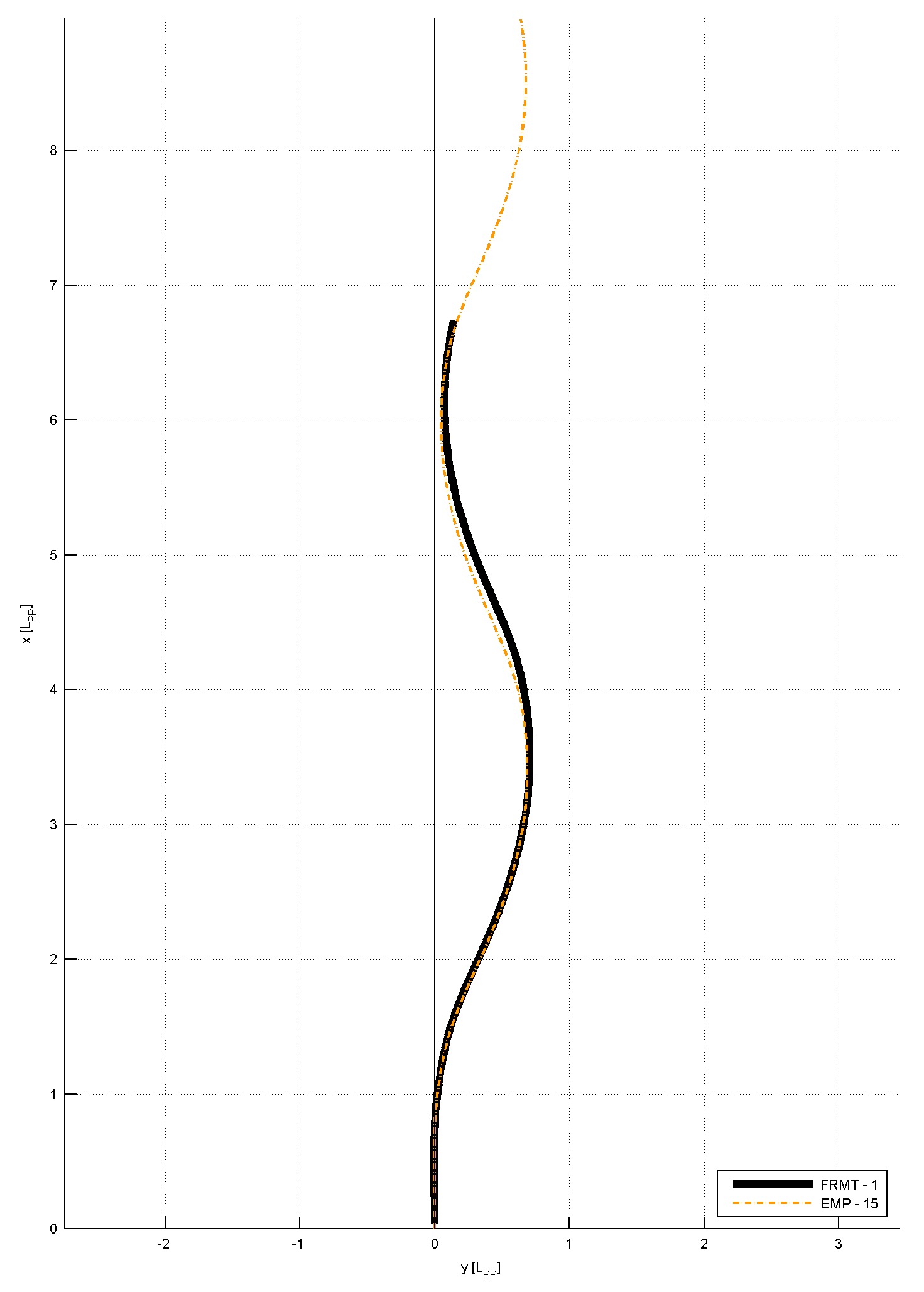Figure 4: Example trajectory of Case 5.2.2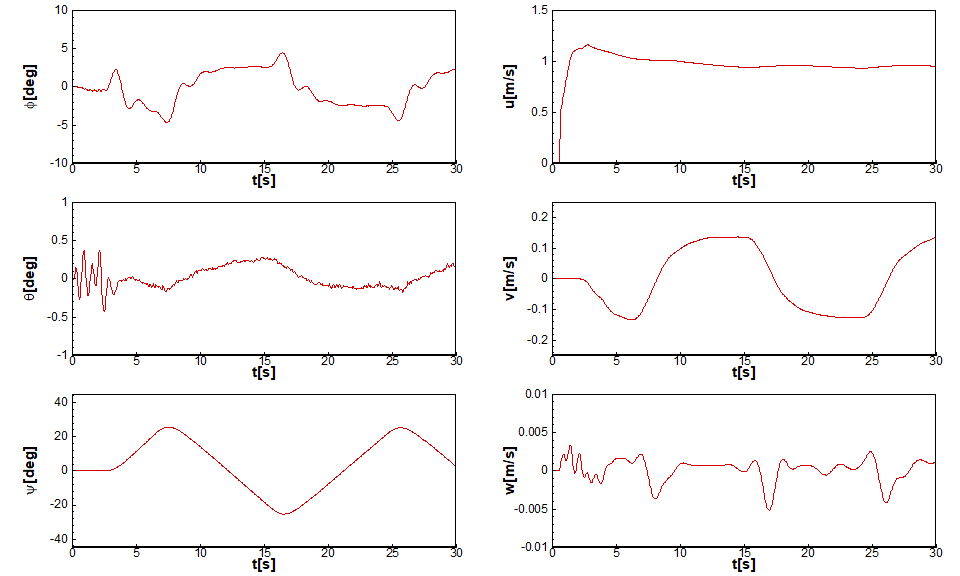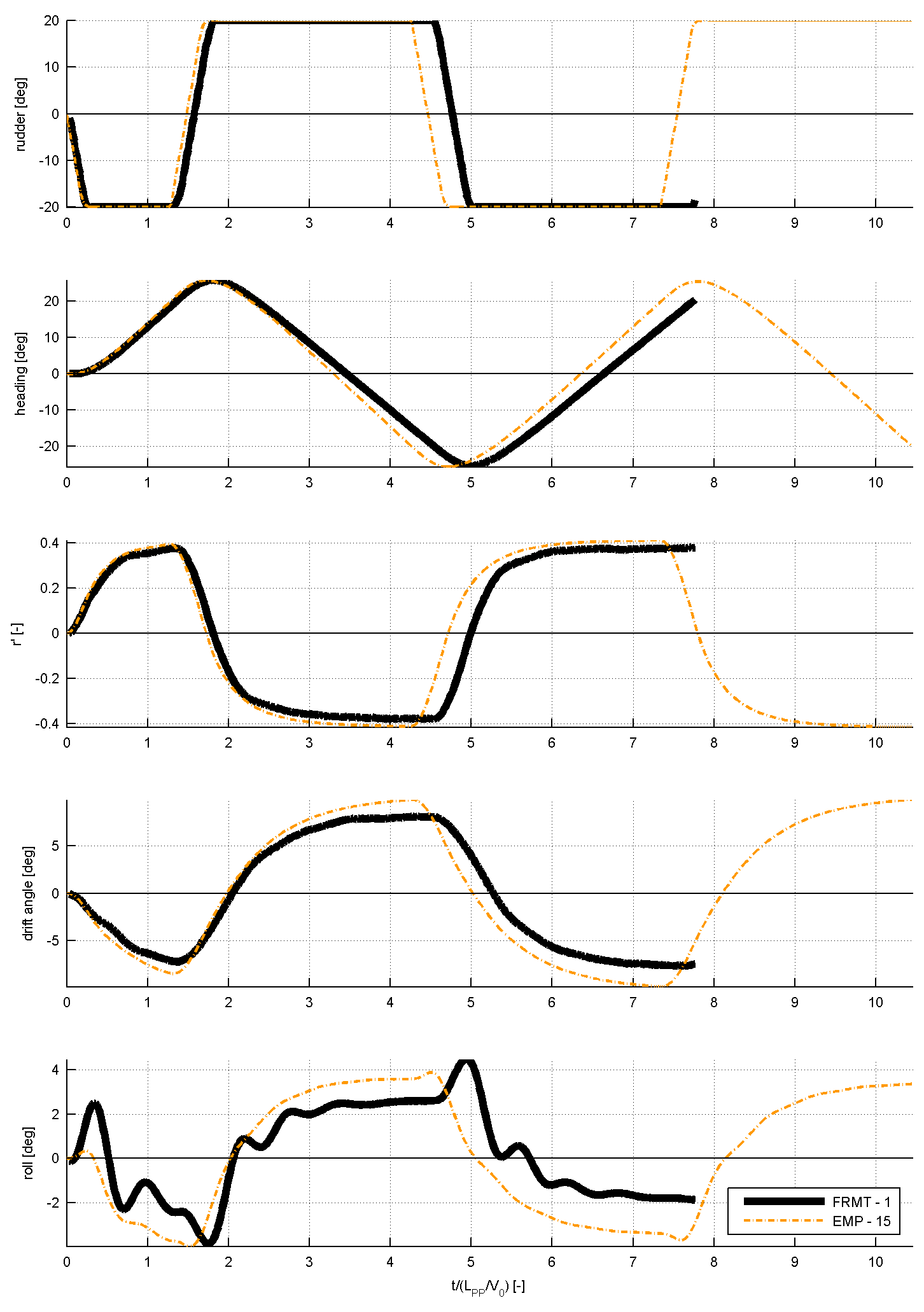Figure 5: Time traces of several signals for Case 5.2.2

##### Table 4: Comparison values for Case 5.2.2
Category Unit Validation Variables
Overshoot angles [deg] 1st overshoot angle (ψOS1)
[deg] 2nd overshoot angle (ψOS2)
[deg] 3nd overshoot angle (ψOS3)
[s] Time of 2nd rudder execute (t2)
[s] Time of 3rd rudder execute (t3)
[s] Time of 4th rudder execute (t4)
[s] Time of 5th rudder execute (t5)
Times [s] Period (time between the 4th and 2nd rudder execute = t4-t2)
[s] 1st overshoot time (tOS1)
[s] 2nd overshoot time (tOS2)
[s] 3rd overshoot time (tOS3)
Maximum yaw rate [deg/s] Maximum yaw rate during 1st overshoot between t2 and t3 (r1)
[deg/s] Maximum yaw rate during 2nd overshoot between t3 and t4 (r2)
[deg/s] Maximum yaw rate during 3rd overshoot between t4 and t5 (r3)
Maximum drift angle [deg] Maximum drift angle during 1st overshoot between t2 and t31)
[deg] Maximum drift angle during 2nd overshoot between t3 and t42)
[deg] Maximum drift angle during 3rd overshoot between t4 and t53)
Maximum roll angle [deg] Maximum roll angle during 1st overshoot between t2 and t31)
[deg] Maximum roll angle during 2nd overshoot between t3 and t42)
[deg] Maximum roll angle during 3rd overshoot between t4 and t53)
##### Table 5: EFD values summary for Case 5.2.2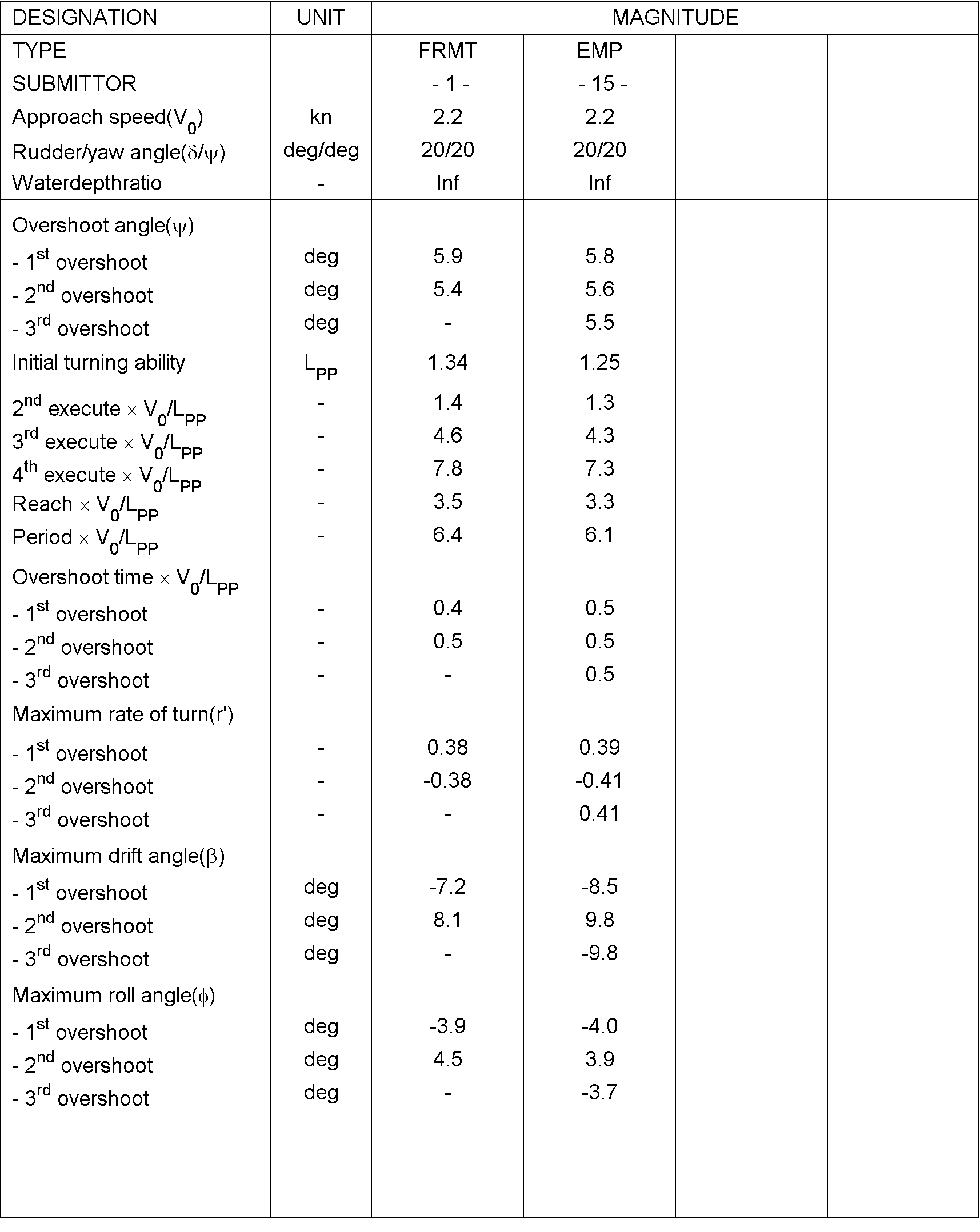## 7. Elaborated example for case 5.2.3: 35° turning circle manoeuvre to portside

For case 5.2.3, the time traces and a bird-eye view will be plotted for comparison as Figure 6 and this will look as displayed in Figure 7. From these time traces, the typical characteristics will be determined. These characteristics will be the comparison variables. Table 6 gives these characteristics. The bold variables are the primary validation variable, the non-bold are the secondary validation variables.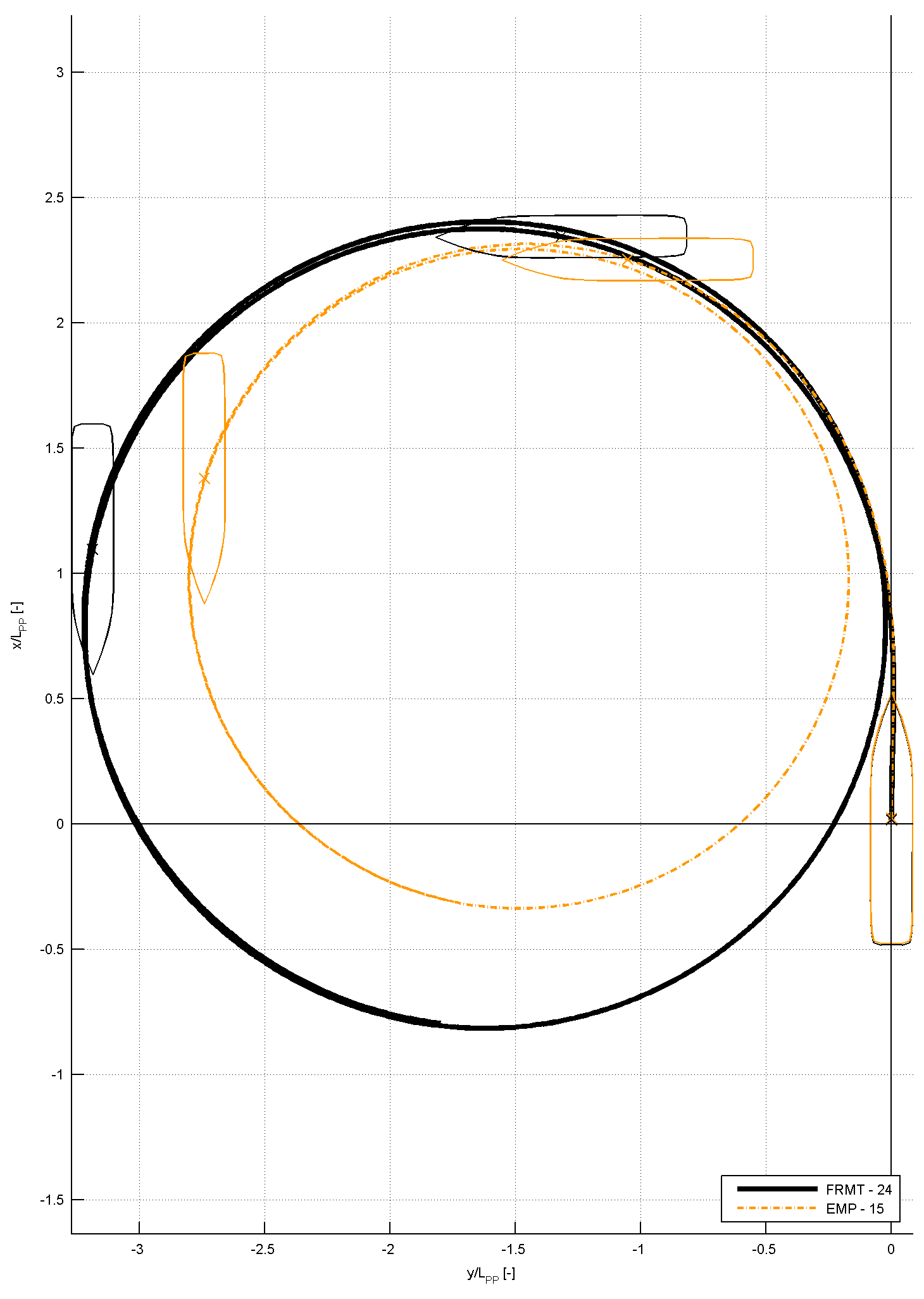Figure 6: Example trajectory of Case 5.2.3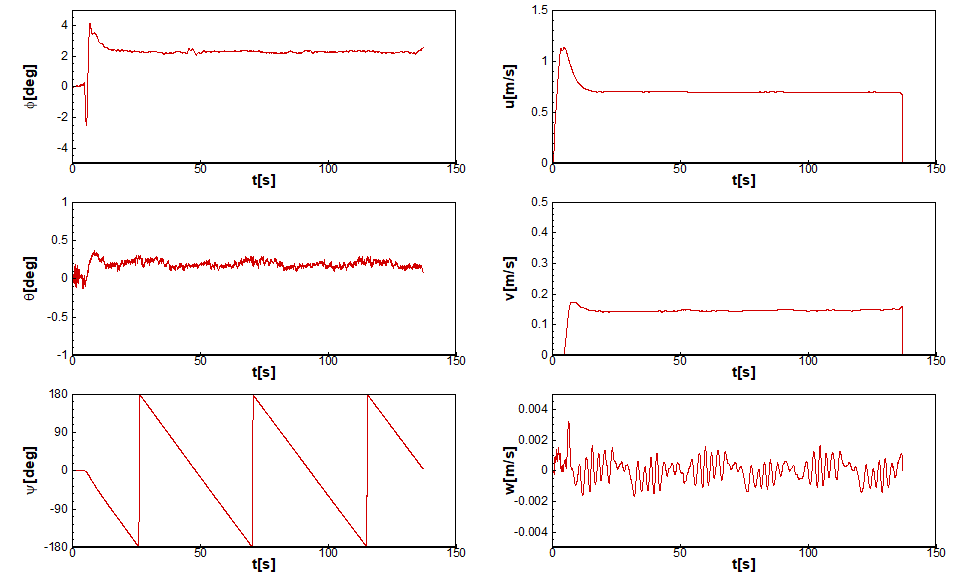Figure 7: Time traces and bird-eye views of Case 5.2.3

##### Table 6: Comparison values for the 35° turning circle manoeuvre
Category Unit Validation Variables
[-] Tactical diameter / LPP (TD/LPP)
[-] Transfer / LPP (Tr/LPP)
Times [s] Time to reach 90° (t90°)
Yaw rate [deg/s] Maximum yaw rate (rmax)
[deg/s] Average yaw rate in the constant part of the turn (rC)
Speed [m/s] Speed in the steady part of the turn (UC)
Roll angles [deg] Maximum inward roll angle (ϕinmax)
[deg] Maximum outward roll angle (ϕoutmax)
[deg] Roll angle in the constant part of the turn (ϕc)
Non-dimensional values [-] Non-dimensional yaw rate in the constant part of the turn (r’)
[deg] Drift angle in the constant part of the turn (βC)
[-] Pivot point (-sin(βC)/r’)
[-] Speed ratio (UC/U0)
##### Table 7: EFD values summary for Case 5.2.3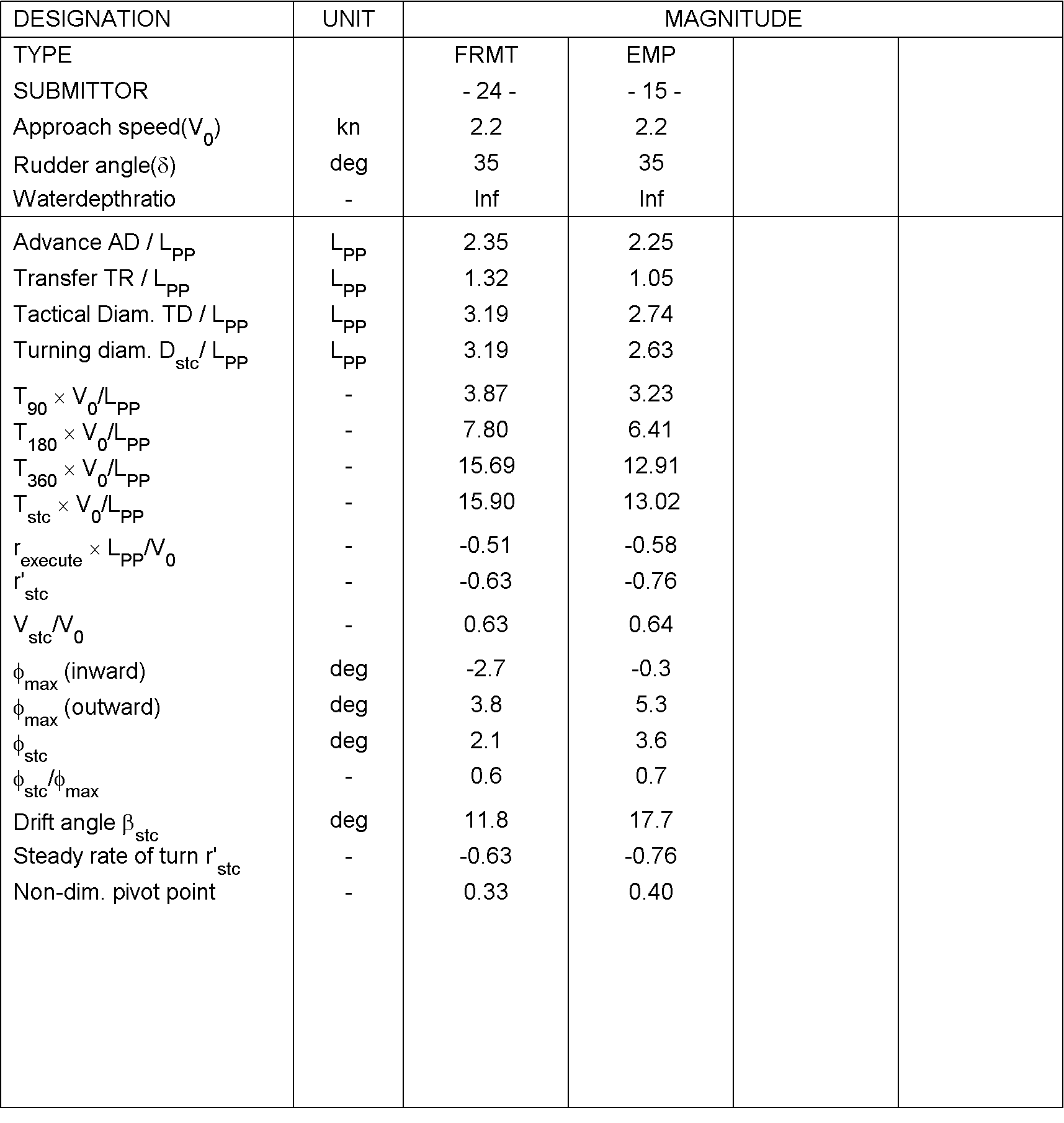(34103) (171 Jang-dong) 32 1312beon-gil, Yuseong-daero, Yuseong-gu, Daejeon, KOREA